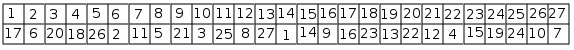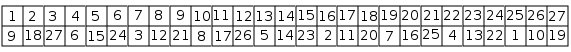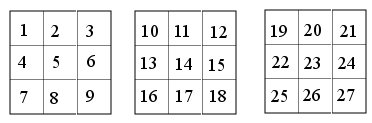# CAIA surprised me Part III The results

After finding symmetries, CAIA tries to find solutions to the magic cube problem, applying rules that enrich its formulation.

One of these rules states that, when there is a bijection between a set of unknowns and a set of values, the sum of the unknowns equals the sum of the values. Therefore, CAIA adds the constraint:

F(1)+F(2)+f(3)+…..+F(26)+F(27)=1+2+3+…..+26+27=378

When it substitutes the first three constraints in the preceding one, it sees that 3*VAL=378, so:

VAL=126

Using this value, and after many substitutions in the constraints, it finds several constraints with four unknowns, such as:

F(5)+F(23)=F(13)+F(15)

Later, it discovers a constraint with three unknowns:

F(5)+F(14)+F(23) = 42

Using the symmetries, it immediately adds two other constraints with three unknowns:

F(11)+F(14)+F(17) = 42

F(13)+F(14)+F(15) = 42

All the other symmetries lead to one of the three preceding constraints.

Finally, it happens that it has no more rule to apply. CAIA can choose between two possibilities:

1. It chooses an unknown, and it examines what happens when it substitutes it by one of its possible value. It carries on this task, instantiating an unknown, then generating new constraints, until it finds a contradiction or a solution. In both cases, it backtracks, instantiating the last unknown by the following possible value. CAIA is not very fast: it finds a solution every three seconds, taking into account that a new solution immediately leads to 47 other solutions, using the symmetries.

2. It writes a combinatorial program, cleverly using the constraints that it has found. This C program has 280 lines. CAIA compiles it, and executes it. Then, it finds 130 solutions every second.

In both cases, it chooses to begin backtracking with F(14), the small cube in the center of the large one. There are many solutions for each of the 27 possible values for F(14).

Here is one of these solutions:Its success is mainly due to its analysis of the problem formulation: it finds symmetries, and collects useful new constraints, particularly the three constraints with three unknowns. I did not know them myself, I had only found constraints with four unknowns. Naturally, when one knows that they exist, it is easy to prove them.

A human researcher, who would specifically solve this problem, could realize a more efficient system. However, the intelligence would be in his head, not in his system.

I wanted to compare CAIA with other problem solvers. Francis Sourd kindly gave this problem to two of the best general solvers for problems defined by constraints. ILOG Solver did not find a solution in a reasonable time, while ILOG CEPLEX found the first one after 14 hours. Incidentally, it shows that CEPLEX is excellent: the search space is enormous when it is impossible to manipulate the formulation of the problem. Therefore, it does not know the value of VAL, and all of its constraints have 10 unknowns.

CAIA solved this problem in a way that I did not know. As Pégoud with his plane, we must let our systems fend for themselves!

I doubt that, at the moment, there exists a general system that could approach the performances of CAIA for this problem, when it is placed in the same conditions: knowing only to find a bijection satisfying the 15 constraints given in part I. If I am wrong, I would be very interested in knowing more on this system.

# CAIA surprised me Part II The symmetries

For the cube problem described in the preceding post, CAIA begins with looking for symmetries in the problem formulation. What is a symmetry? For Richard Feynman: « A thing is symmetrical if there is something we can do to it so that after we have done it, it looks the same as it did before. »

There are many kinds of symmetries. CAIA only tries to find one of them: a permutation of the unknowns leads to the same set of constraints when each unknown is replaced by the corresponding one.

To do this, I defined a combinatorial problem: finding all the permutations of the unknowns that satisfy the preceding condition. This problem is given in the formalism used for all the combinatorial problems solved by CAIA. The interest of the bootstrap clearly appears here: knowing how to solve combinatorial problems allows us to solve problems necessary for solving combinatorial problems. For every new problem, CAIA solves a preliminary problem: finding which symmetries exist. Its data are the initial constraints of the main problem.

We are not restricted to the case where each constraint C is transformed in itself by the permutation, such as when one permutes A and B in the constraint A+B>0. Usually, most constraints are transformed into other ones.

For the cube problem, CAIA finds 47 solutions: for each solution that it finds, it can immediately add 47 new solutions.

One of these solutions is the following permutation:All the classical geometrical symmetries are among these 47 solutions: to the center, to the mean planes, etc. However, it found them from the constraints: it does not know geometry; the method can be used for problems where there is no geometrical interpretation. For instance, if unknowns A and B appear only in the constraint A+B=1,000,000 exchanging A and B gives the same constraint. Naturally, CAIA can often add new constraints for avoiding to generate a solution and its symmetrical ones. In the preceding example, if the values of A and B are different, adding constraint A<B will remove all the symmetrical solutions.

For three main reasons, CAIA needs finding symmetries:

1. This decreases the size of the search space, because constraints are added to prevent finding symmetrical solutions.

2. This increases the speed of the resolution process: for every new solution, one can immediately generate all its symmetrical solutions. For the cube, the system is 48 times faster once it has found the symmetries.

3. It is easier to create constraints: for each new constraint, CAIA adds all the constraints that are obtained by symmetry. Let us notice that it does not generate 47 other constraints for each constraint: as few unknowns are in a constraint, many of the constraints generated in that way are identical.

If one believes to help the system by adding constraints to the initial formulation, this is a mistake: this may hide some symmetries. For instance, one may be proud to have proven the constraint F(17)+F(11)=F(5)+F(23). Unfortunately, adding this constraint in the initial formulation will remove most of the symmetries, including the one given above. Indeed, the constraint that would be created with this symmetry is F(11)+F(17)=F(15)+F(13); it is not among the initial constraints of this new formulation. As it often happens, when one has a clever system, it is not good to try to help it: one may complicate its task. Let us trust CAIA to do its job.

Finally, for me, the main interest of finding symmetries is to show concretely that bootstrapping is possible, and can be very useful: realizing a solver for problems defined by a set of constraints improves the solver itself.

In the next post, we will see how CAIA uses these symmetries for solving the magic cube problem.

# CAIA surprised me Part I

An AI researcher can always arrange his system so that it can find a solution that he already knows. To do that, he chooses a formulation well adapted to this solution, he includes all the methods necessary for this solution, and he indicates to avoid the roads that do not lead to the right direction. For this reason, it is always interesting to see a system finding a solution unknown by its author.

We have already seen how ALICE, developed by Jean-Louis Laurière, had immediately solved a problem proposed by professor Schutzenberger. I will now describe how CAIA has solved a problem better than I would have done.

Let us consider a kind of magic cube: the goal is to find a bijection F between the 27 small cubes that are in a 3x3x3 large cube, and the integers between 1 and 27. The sums of the planes containing nine cubes must have the same value VAL. There are 15 such planes: 3 horizontal, 6 vertical, and 6 diagonal. The small cubes are numbered in the following way, the first array is for the upper face, and the third one for the lower face, as shown below:The three constraints for the horizontal planes are:

F(1)+F(2)+F(3)+F(4)+F(5)+F(6)+F(7)+F(8)+F(9) = VAL

F(10)+F(11)+F(12)+F(13)+F(14)+F(15)+F(16)+F(17)+F(18)=VAL

F(19)+F(20)+F(21)+F(22)+F(23)+F(24)+F(25)+F(26)+F(27)=VAL

The six constraints for the vertical planes:

F(1)+F(2)+F(3)+F(10)+F(11)+F(12)+F(19)+F(20)+F(21)=VAL

F(4)+F(5)+F(6)+F(13)+F(14)+F(15)+F(22)+F(23)+F(24)=VAL

F(7)+F(8)+F(9)+F(16)+F(17)+F(18)+F(25)+F(26)+F(27)=VAL

F(1)+F(4)+F(7)+F(10)+F(13)+F(16)+F(19)+F(22)+F(25)=VAL

F(2)+F(5)+F(8)+F(11)+F(14)+F(17)+F(20)+F(23)+F(26)=VAL

F(3)+F(6)+F(9)+F(12)+F(15)+F(18)+F(21)+F(24)+F(27) = VAL

The last six constraints are for the diagonal planes:

F(1)+F(2)+F(3)+F(13)+F(14)+F(15)+F(25)+F(26)+F(27)=VAL

F(7)+F(8)+F(9)+F(13)+F(14)+F(15)+F(19)+F(20)+F(21)=VAL

F(1)+F(4)+F(7)+F(11)+F(14)+F(17)+F(21)+F(24)+F(27) = VAL

F(3)+F(6)+F(9)+F(11)+F(14)+F(17)+F(19)+F(22)+F(25)=VAL

F(1)+F(5)+F(9)+F(10)+F(14)+F(18)+F(19)+F(23)+F(27)=VAL

F(3)+F(5)+F(7)+F(12)+F(14)+F(16)+F(21)+F(23)+F(25)=VAL

In this old version of CAIA, I have indicated that the value of VAL was between 1 and 300. This was a very small aid; in the future versions, telling that VAL is positive will be enough.

I have already drawn attention to the importance of the problem formulation. To sum up, CAIA knows that it must find a bijection of 27 unknowns on the set of integers from 1 to 27, and the value of the positive unknown VAL, less than 300, such that the 15 preceding constraints are satisfied.

One could think that this is an easy problem, since it is under-constrained: there are 28 unknowns and only 15 constraints, plus the fact that one has a bijection. It has millions of solutions. However, the search tree is very large since every F has 27 possible values, and 300 for VAL. Moreover, each constraint contains 10 unknowns, and one must instantiate 9 of them to get a value for the tenth: many instantiations have to be made before one sees a contradiction. From this point of view, the problem is extremely difficult to solve: there are many solutions, but they are hidden in billions of possible instantiations for the unknowns.

We will see in the following posts how CAIA solved this problem, beginning with the discovery of its symmetries.